# Publications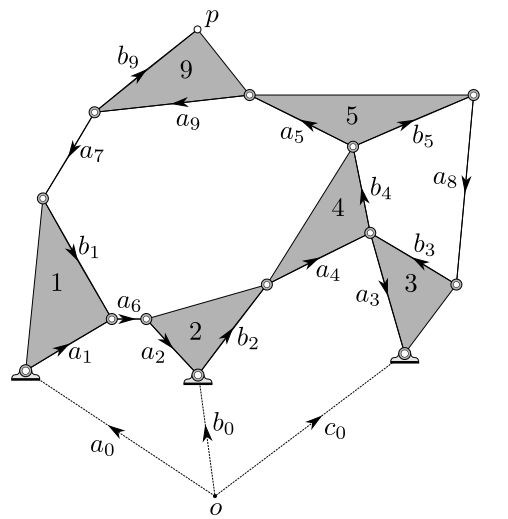Ten-bar planar mechanism that we derive a cognate of.

A general method for constructing planar cognate mechanisms.
Samantha N. Sherman, Jonathan D. Hauenstein, and Charles W. Wampler. Submitted for publication Sept. 2020. (pdf)
Abstract: Cognate linkages are mechanisms that share the same mo- tion, a property that can be useful in mechanical design. This paper treats planar curve cognates, that is, planar mecha- nisms whose tracing point draws the same curve, as well as coupler cognates and timed curve cognates. The purpose of this article is to show how cognates can be easily understood and constructed by using kinematic equations. The method reproduces all the known results for four-bars and six-bars, and we show by example that it also can be used to construct eight-bar and ten-bar cognates.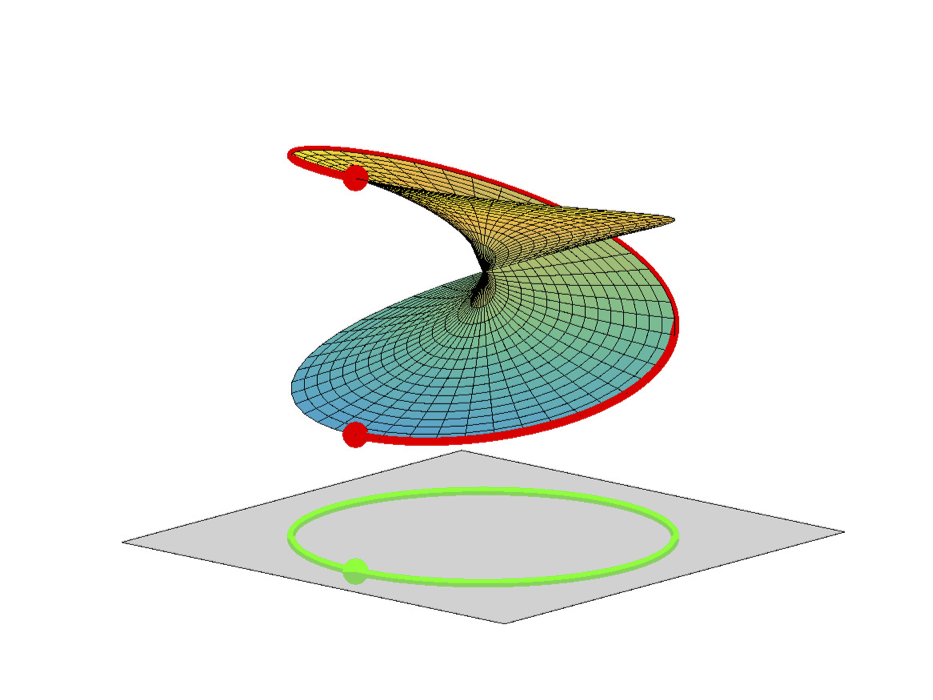Example of monodromy.

Using monodromy to statistically estimate the number of solutions.
Jonathan D. Hauenstein and Samantha N. Sherman. To appear in Springer’s Proceedings in Advanced Robotics. (pdf, arXiv:2005.00327, computations page)
Abstract: Synthesis problems for linkages in kinematics often yield large structured parameterized polynomial systems which generically have far fewer solutions than traditional upper bounds would suggest. This paper describes statistical models for estimating the generic number of solutions of such parameterized polynomial systems. The new approach extends previous work on success ratios of parameter homotopies to using monodromy loops as well as the addition of a trace test that provides a stopping criterion for validating that all solutions have been found. Several examples are presented demonstrating the method including Watt I six bar motion generation problems.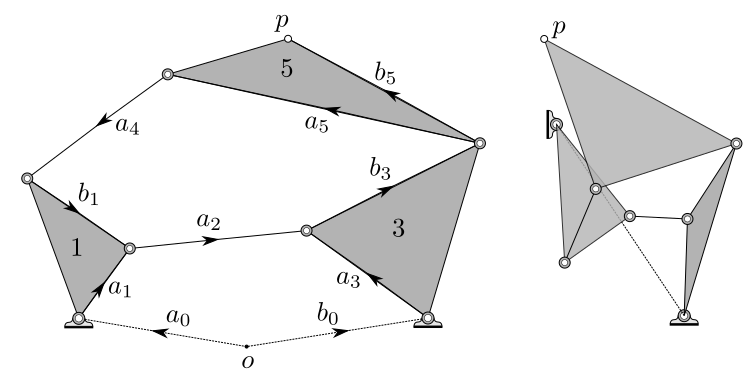A Stephenson I mechanism (left) and its curve cognate (left).

Samantha N. Sherman, Jonathan D. Hauenstein, and Charles W. Wampler. To appear in Proceedings of the ASME 2020 International Design Engineering Technical Conferences and Computers and Information in Engineering Conference(pdf)
Abstract: Cognate linkages are mechanisms that share the same motion, a property that can be useful in mechanical design. This paper treats planar curve cognates, that is, planar mechanisms whose tracing point draws the same curve. While Roberts cognates for planar four-bars are relatively simple to understand from a geometric drawing, the same cannot be said for planar six-bar cognates, especially the intricate diagrams Dijksman presented in cataloging all the known six-bar curve cognates. The purpose of this article is to show how the six-bar cognates can be easily understood using kinematic equations written using complex vectors, giving a simple method for generating these cognates as alternatives in a mechanical design. The simplicity of the approach enables the derivation of cognates for eight-bars and possibly beyond.

Computing sparse polynomials via witness sets.
Jonathan D. Hauenstein, Laura Matusevich, Chris Peterson, and Samantha N. Sherman. In preparation. (pdf)
Abstract: Sparse polynomials that vanish on algebraic sets are preferred in many computations since they are easy to evaluate and often arise from underlying structure. For example, a monomial vanishes on an algebraic set if and only if the algebraic set is contained in the union of the coordinate hyperplanes. This paper considers computing sparse polynomials that vanish on an algebraic set which is represented by a witness set. Our approach relies on using numerical homotopy methods to sample points on the algebraic set along with incorporating multiplicity information using Macaulay dual spaces. If the algebraic set is defined by polynomials with rational coefficients, exactness recovery such as lattice based methods can be used to find exact representations of the sparse polynomials and Gröbner basis techniques can be used to certify correctness. Several examples are presented demonstrating the approach.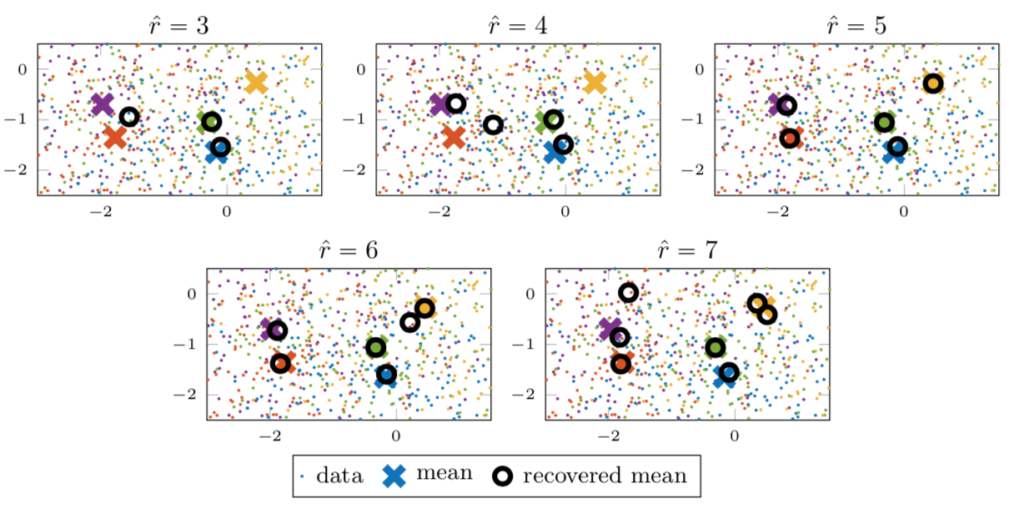Experiment recovering means from a Gaussian mixture model using our implicit symmetric tensor decomposition method.

Estimating Higher-Order Moments Using Symmetric Tensor Decomposition.
Samantha N. Sherman and Tamara G. Kolda. SIAM Journal on Matrix Analysis and Applications, June 2020, accepted for publication.
( pdf, poster, arXiv:1911.03813)
Abstract: We consider the problem of decomposing higher-order moment tensors, i.e., the sum of symmetric outer products of data vectors. Such a decomposition can be used to estimate the means in a Gaussian mixture model and for other applications in machine learning. The dth-order empirical moment tensor of a set of p observations of n variables is a symmetric d-way tensor. Our goal is to find a low-rank tensor approximation comprising rp symmetric outer products. The challenge is that forming the empirical moment tensors costs O(pnd) operations and O(nd) storage, which may be prohibitively expensive; additionally, the algorithm to compute the low-rank approximation costs O(nd) per iteration. Our contribution is avoiding formation of the moment tensor, computing the low-rank tensor approximation of the moment tensor implicitly using O(pnr) operations per iteration and no extra memory. This advance opens the door to more applications of higher-order moments since they can now be efficiently computed. We present numerical evidence of the computational savings and show an example of estimating the means for higher-order moments.Torus with Hamming minimizers to point a shown as the red stars.

Solving critical point conditions for the Hamming and taxicab distances to solution sets of polynomial equations.
Danielle A. Brake, Noah S. Daleo, Jonathan D. Hauenstein, and Samantha N. Sherman.  2019 21st International Symposium on Symbolic and Numeric Algorithms for Scientific Computing, pp. 51-58.
(pdf)
Abstract: Minimizing the Euclidean distance (l2 -norm) from a given point to the solution set of a given system of polynomial equations can be accomplished via critical point techniques. This article extends critical point techniques to minimization with respect to Hamming distance (l0-“norm”) and taxicab distance (l1 -norm). Numerical algebraic geometric techniques are derived for computing a finite set of real points satisfying the polynomial equations which contains a global minimizer. Several examples are used to demonstrate the new techniques.

Certifying reality of projections.
Jonathan D. Hauenstein, Avinash Kulkarni, Emre C. Sertoz, and Samantha N. Sherman. LNCS, 10931, 200-208, 2018.
(pdf, computations page, arXiv:1804.02707)
Abstract: Computational tools in numerical algebraic geometry can be used to numerically approximate solutions to a system of polynomial equations. If the system is well-constrained (i.e., square), Newton’s method is locally quadratically convergent near each nonsingular solution. In such cases, Smale’s alpha theory can be used to certify that a given point is in the quadratic convergence basin of some solution. This was extended to certifiably determine the reality of the corresponding solution when the polynomial system is real. Using the theory of Newton-invariant sets, we certifiably decide the reality of projections of solutions. We apply this method to certifiably count the number of real and totally real tritangent planes for instances of curves of genus 4.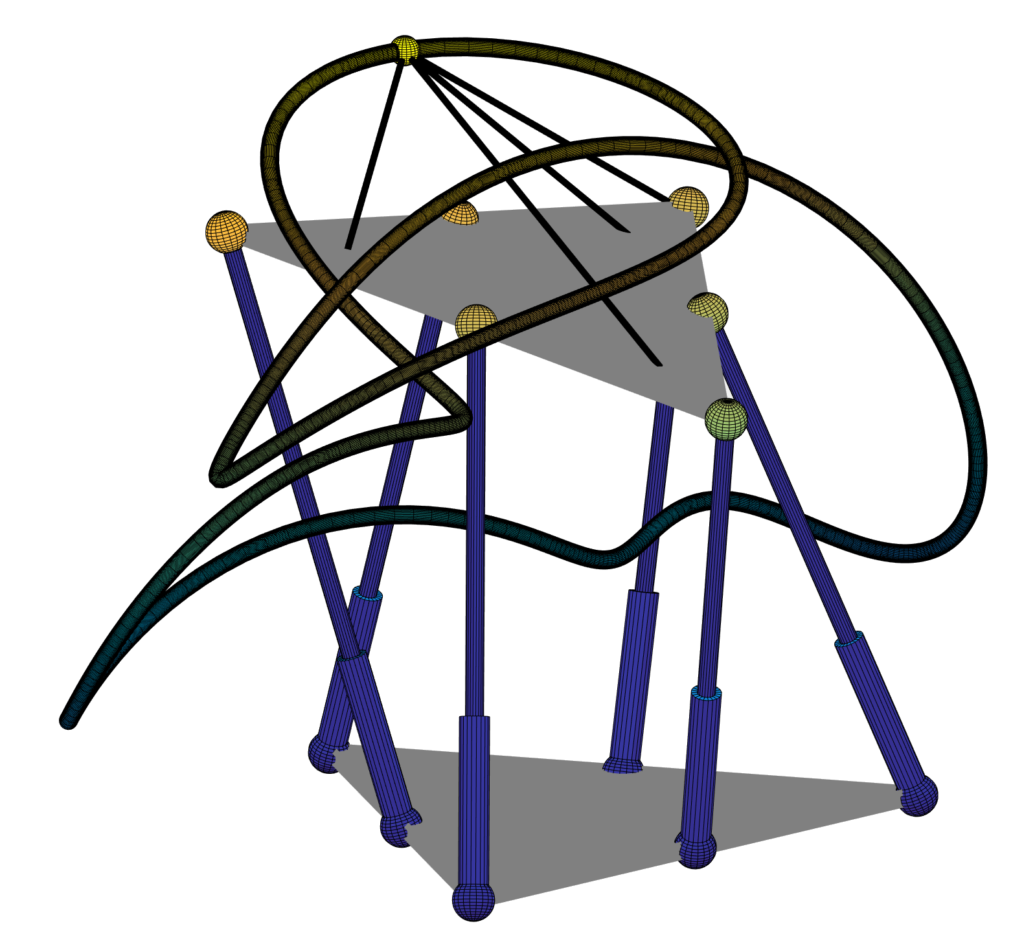Stewart-Gough platform and its motion curve.

Exceptional Stewart-Gough platforms, Segre embeddings, and the special Euclidian group.
Jonathan D. Hauenstein, Samantha N. Sherman, and Charles W. Wampler.  SIAM Journal on Applied Algebraic Geometry, 2(1), 179-205, 2018. (pdf, computations page, poster)

Abstract: Stewart-Gough platforms are mechanisms which consist of two rigid objects, a base and a platform, connected by six legs via spherical joints. For fixed leg lengths, a generic Stewart-Gough platform is rigid with 40 assembly configurations (over the complex numbers) while exceptional Stewart-Gough platforms have infinitely many assembly configurations and thus have self-motion. We define a family of exceptional Stewart-Gough platforms called Segre-dependent Stewart-Gough platforms which arise from a linear dependency of point-pairs under the Segre embedding and compute an irreducible decomposition of this family. We also consider Stewart-Gough platforms which move with two degrees of freedom. Since the Segre embedding arises from a representation of the special Euclidean group in three dimensions which has degree 40, we consider the special Euclidean group in other dimensions and compute spatial Stewart-Gough platforms that move in 4-dimensional space.